# Positive cone

A subsetof a real vector spacesatisfying the following conditions:

1) if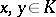and, then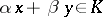;

2).

A positive cone defines a pre-order inby putting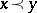if. (This pre-order is compatible with the vector space operations.)

Letbe a Banach space. The coneis a closed reproducing positive cone if for all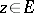there aresuch that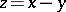. In that case there is a constantindependent ofsuch that there always exist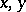such thatwith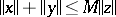. A solid positive cone, i.e. one having interior points, is reproducing.

Let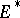be the dual of the Banach space. If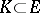is a closed reproducing positive cone, then the set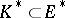of positive functionals (with respect to the positive cone, i.e. thosesuch that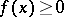for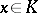) is also a positive cone (this is the so-called conjugate cone). The positive conecan be recovered from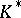, namely:Ifis a solid positive cone, then its interior coincides withA cone in the Banach spaceis called normal if one can find aso thatfor. A positive cone is normal if and only if the conjugate coneis reproducing. Ifis a reproducing cone, then the conjugate coneis normal.

A coneis called a lattice cone if each pair of elementshas a least upper bound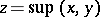, i.e.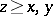and for any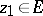it follows fromthat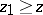. If a positive cone is regular and lattice, then any countable bounded subset has a least upper bound.